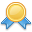# DynDeveloper.com

 Table Edits Forum Articles000
sosume 10/14/2012 1:13:29 PM

## Batch Transaction

I need an example of using eConnect and inserting a payable batch transaction with a header and distribution.

I have been able to get all of the serialization working but, I cannot figure out the steps to take to get the xml to import.

I am getting "Sequence contains no elements"

Here is my code so far:

`    ``Dim sFileName As String = Environment.GetFolderPath(Environment.SpecialFolder.Desktop) & "\Frase.xml"`
`    ``Dim oBatchHdr As New taCreateUpdateBatchHeaderRcd`
`    ``Dim oMemoryStream As New MemoryStream`
`    ``Dim oSerializer As New XmlSerializer(GetType(eConnectType))`
`    ``Dim oPymtDistribution As New taPMDistribution_ItemsTaPMDistribution`
`    ``Dim oPaymentTransaction As New taPMTransactionInsert`
`    ``Dim oConnect As New eConnectType`
`    ``Dim sBatchNumber As String = "EXP_RPT_" & Format(Now, "mmddyy")`
`    ``Dim oTransType As New PMTransactionType`

`    ``With oBatchHdr`
`        ``.APPROVL =`
`        ``.BACHFREQ = 1`
`        ``.BACHNUMB = sBatchNumber`
`        ``.BCHCOMNT = "Automated Entry"`
`        ``.BCHSOURC = "No Clue Source"`
`        ``.BACHFREQSpecified = True`
`        ``.DOCAMT = 25.0`
`        ``.NUMOFTRX = 1`
`        ``.ORIGIN = 1`
`        ``.SERIES = 4`
`        ``'.APPRVLDT =`
`        ``'.APRVLUSERID =`
`        ``'.BRKDNALL =`
`        ``'.CHEKBKID =`
`        ``'.CNTRLTOT =`
`        ``'.CNTRLTOTSpecified =`
`        ``'.CNTRLTRX =`
`        ``'.CNTRLTRXSpecified =`
`        ``'.EFTFileFormat =`
`        ``'.GLPOSTDT =`
`        ``'.MSCBDINC =`
`        ``'.MSCBDINCSpecified =`
`        ``'.PmtMethod =`
`        ``'.POSTTOGL =`
`        ``'.RECPSTGS =`
`        ``'.RECPSTGSSpecified =`
`        ``'.RequesterTrx =`
`        ``'.TRXSOURC = `
`        ``'.USERID = `
`        ``'.USRDEFND1 = `
`        ``'.USRDEFND2 =`
`        ``'.USRDEFND3 =`
`        ``'.USRDEFND4 =`
`        ``'.USRDEFND5 =`
`    ``End With`

`    ``With oPaymentTransaction`
`        ``.BACHNUMB = sBatchNumber`
`        ``.DOCAMNT = 25.0`
`        ``.DOCDATE = Now`
`        ``.DOCNUMBR = "ABC-123"`
`        ``.DOCTYPE = 1`
`        ``.VENDORID = "FRASE-001"`
`        ``'    .APDSTKAM =`
`        ``'    .BatchCHEKBKID =`
`        ``'    .CAMCBKID =`
`        ``'    .CAMPMTNM =`
`        ``'    .CAMPYNBR =`
`        ``'    .CAMTDATE =`
`        ``'    .CARDNAME =`
`        ``'    .CASHAMNT =`
`        ``'    .CCAMPYNM =`
`        ``'    .CCRCTNUM =`
`        ``'    .CDOCNMBR =`
`        ``'    .CHAMCBID =`
`        ``'    .CHEKAMNT =`
`        ``'    .CHEKBKID =`
`        ``'    .CHEKDATE =`
`        ``'    .CHEKNMBR =`
`        ``'    .CHRGAMNT =`
`        ``'    .CRCARDDT =`
`        ``'    .CRCRDAMT =`
`        ``.CREATEDIST = 0`
`        ``'    .CURNCYID =`
`        ``'    .DATELMTS =`
`        ``'    .DISAMTAV =`
`        ``'    .DISAMTAVSpecified =`
`        ``'    .DISCDATE =`
`        ``'    .DISTKNAM =`
`        ``'    .DSCDLRAM =`
`        ``'    .DSCDLRAMSpecified =`
`        ``'    .DUEDATE =`
`        ``'    .DYSTINCR =`
`        ``'    .EXCHDATE =`
`        ``'    .EXGTBDSC =`
`        ``'    .EXPNDATE =`
`        ``'    .EXTBLSRC =`
`        ``'    .FRTAMNT =`
`        ``'    .FRTSCHID =`
`        ``'    .MDFUSRID =`
`        ``'    .MSCCHAMT =`
`        ``'    .MSCSCHID =`
`        ``'    .PCHSCHID =`
`        ``'    .PORDNMBR =`
`        ``'    .POSTEDDT =`
`        ``'    .PRCHAMNT =`
`        ``'    .PRCTDISC =`
`        ``'    .PRCTDISCSpecified =`
`        ``'    .PRVDSLMT =`
`        ``'    .PSTGDATE =`
`        ``'    .PTDUSRID =`
`        ``'    .PYMTRMID =`
`        ``'    .RATEEXPR =`
`        ``'    .RATETPID =`
`        ``'    .RATEVARC =`
`        ``'    .RequesterTrx =`
`        ``'    .RTCLCMTD =`
`        ``'    .SHIPMTHD =`
`        ``'    .Tax_Date =`
`        ``'    .TAXAMNT =`
`        ``'    .TAXSCHID =`
`        ``'    .TIME1 =`
`        ``'    .TEN99AMNT =`
`        ``'    .TIME1 =`
`        ``'    .TRDISAMT =`
`        ``'    .TRXDSCRN =`
`        ``'    .TRXDTDEF =`
`        ``'    .USRDEFND1 =`
`        ``'    .USRDEFND2 =`
`        ``'    .USRDEFND3 = `
`        ``'    .USRDEFND4 = `
`        ``'    .USRDEFND5 = `
`        ``'    .VADCDTRO = `
`        ``'    .VADDCDPR = `
`        ``'    .VCHNUMWK = `
`        ``'    .XCHGRATE = `
`    ``End With`

`    ``With oPymtDistribution`
`        ``.ACTINDX =`
`        ``.ACTNUMST = "000-1100-00"`
`        ``.CRDTAMNT = 12`
`        ``.DEBITAMT = 0`
`        ``'.DistRef =`
`        ``'.DISTTYPE = 6`
`        ``.DOCTYPE = 1`
`        ``'.DSTSQNUM = `
`        ``.VCHRNMBR = "VCHR-110"`
`        ``.VENDORID = "FRASE-001"`
`    ``End With`

`    ``oTransType.taPMTransactionInsert = oPaymentTransaction`
`    ``'oConnect.PMTransactionType(0) = oBatchHdr`
`    ``ReDim oConnect.PMTransactionType(0)`
`    ``ReDim oTransType.taPMDistribution_Items(0)`

`    ``oTransType.taPMDistribution_Items(0) = oPymtDistribution`
`    ``oConnect.PMTransactionType(0) = oTransType`

`    ``Dim fs As New FileStream(sFileName, FileMode.Create)`
`    ``Dim writer As New XmlTextWriter(fs, New UTF8Encoding)`
`    ``'Serialize using the XmlTextWriter to the file`
`    ``Dim serializer As New XmlSerializer(GetType(eConnectType))`
`    ``serializer.Serialize(writer, oConnect)`
`    ``writer.Close()`

`    ``Dim oXMLDoc As New Xml.XmlDocument`
`    ``Dim bRetCode As Boolean`

`    ``oXMLDoc.Load(sFileName.ToString)`

`    ``Dim oConnMethod As New Microsoft.Dynamics.GP.eConnect.eConnectMethods`

`    ``bRetCode = oConnMethod.CreateTransactionEntity("Data Source=localhost;Integrated Security=SSPI;Persist Security Info=False;Initial Catalog=TWO;Packet Size=4096;", oXMLDoc.OuterXml)`
`Catch ex1 As eConnect.eConnectException`
`    ``MsgBox(ex1.ToString)`
`Catch ex As Exception`
`    ``MsgBox(ex.Message)`
`End Try`

Version: GP 10
Section: .NET Development, Dynamics GP, eConnect

Table Definition Quick Links
All Tables
SOP Tables
RM Tables
GL Tables
POP Tables
HR Tables
PM Tables
UPR Tables
IV Tables
Olympic Tables
3